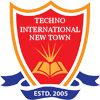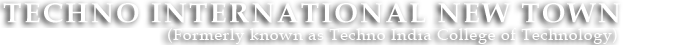#+91 9674112076/2079
••••# DSP AND VLSI LAB

CONTROL SYSTEM LABORATORY

Code:EC-593

List of Experiments:
1. Familiarization with MATLAB control system toolbox and MATLAB-SIMULINK toolbox.
2.Determination of transfer function using block diagram reduction techniques through MATLAB toolbox.
3. Determination of step and impulse response for 1st order & 2nd order system, evaluation of static errors and static error coefficients &calculation of control system specifications for second order system.
4.Determination of root locus using MATLAB control system toolbox for a given 2nd order transfer function
5. Determination of Bode-plot using MATLAB control system toolbox for a given transfer function.
6.Determination of Nyquist Plot using MATLAB control system toolbox for a given transfer function.

DIGITAL SIGNAL PROCESSING LABORATORY

Code: (EC 692)
List of Experiments:

1. Convolution of two sequences using graphical methods and commands - verification of the properties of convolution.
2. Z-transform of various sequences – verification of the properties of Z-transform.
3. Twiddle factors – verification of the properties.
4. DFTs / IDFTs using matrix multiplication and also using commands.
5. Circular convolution of two sequences using graphical methods and commands, differentiation between linear and circular convolutions.
6. Verifications of the different algorithms associated with filtering of long data sequences and Overlap –add & Overlap-save methods.
7. An Innovative Experiment.

VLSI DESIGN LABORATORY

Code: EC792

List of Experiments:

1. Familiarity with Spice simulation tool.
2. Spice Simulation of Inverter, NAND, NOR Gates.
3. Familiarity with EDA tools for VLSI design /FPGA based system design
4. Layouts, Transistors and tools.
5. Standards cell Design.
6. Design of CMOS XOR/XNOR Gates.
7. Design of CMOS Full adder.
8. Design of CMOS Flip flops ( R-S ,D , J-K).
9. Design of 8 bit synchronous Counter.
10. Design of 8 bit bi-directional register with tri-stated input/output bus.
11. Design of a 12 bit CPU with few instructions and implementation and Validation on FPGA.